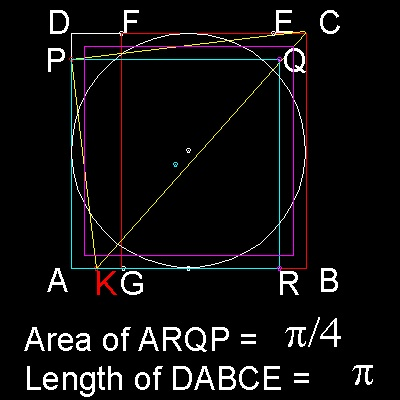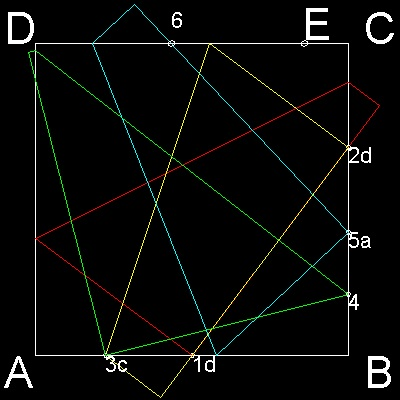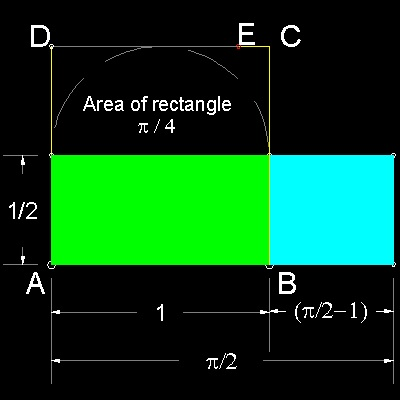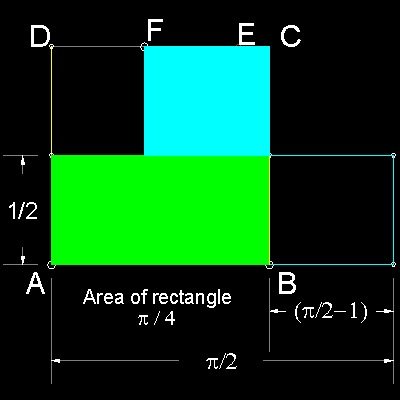Squaring the Circle
Go to   Fun_Math Content Table   Three Famous Problems

### Origami(Paper Folding) Solution--Approximation

#### 1. Create 355/113 exactly on a square paper( by Kazuo Haga )

In the drawing below, ABCD is a square sheet of paper.``` The circle squaring goes through 3 steps. 1. Find a point E on the side CD such that the length of DABCE = 355/113 exact. Use Haga's theorem repeatedly. 2. Make a rectangle with its area = p/4 (FGBC in color red) length FC = p/4 3. Make a square with its area = p/4 (PARQ in color cyan) point K is the mid point of AG. Point P is found by moving corner B using K as pivot until B touches line AD.(in yellow lines) AP is one side of the square. Note that crease line through K is slightly off the point C. ```

***** origami_circle_squarer_desc.dwg ******

#### Step 1: Find point "E"``` 1. Move the corner point D to the mid point of AB ("1d"). Mark the point where CD crosses BC ("2d") This point is at the distance of 1/3 from point C. 2. Move the corner point D to point "2d". This creates point "3c". Distance between B and "3c" is 7/9 . 3. Move point C to "3c". This creates point "4", which is 16/81 from point B. Double this distance (32/81) and creates point "5a". 4. Move corner A to point "5a". This creates point "6" at 64/113 from point C. 5. Take 1/4 of this length from point C. This is point "E". The total length of DABCE is 355/113, which is a very good approximation of p. 355/113 = 3.14159292... p = 3.14159265... ```

******** Haga_circle_squaring_step_1.dwg ********

You can see the process in animation.

To create this drawing and animation:
Then from command line, type Haga_step_1

#### Step 2: Make a rectangle with its area = p/4***** Haga_circle_squaring_step_2_0.dwg ***** ***** Haga_circle_squaring_step_2_1.dwg *****``` Haga_circle_squaring_step_2_0.dwg Area of a circle inscribing the square = p/4 . Haga_circle_squaring_step_2_1.dwg A rectangle , made up of 2 rectangles (green & cyan), has the same area. Haga_circle_squaring_step_2_2.dwg Let F be the mid point of DE. Since DE = 4 - p, FC = 1 - DE/2 = p/2 - 1 So the rectangle in cyan color can be moved up to align with FC. ```

***** Haga_circle_squaring_step_2_2.dwg ********** Haga_circle_squaring_step_2_3.dwg ***** ***** Haga_circle_squaring_step_2_4.dwg *****
 ``` Haga_circle_squaring_step_2_3.dwg Let G be the mid-point of DF, and GH be drawn parallel to DA . Haga_circle_squaring_step_2_4.dwg A part of green rectangle between DA and GH can be moved up to fill space between cyan rectangle and line GH. Now rectangle GHBC has the area = p/4 . ```

To create this drawing :
Then from command line, type Haga_step_2

#### Step 3: Make a square with its area = p/4

The next step is to make a square with the same area as the rectangle. We use Prop. 13 of The Elements, Book VI.************ Euclid_prop_13.dwg ************* *********** Euclid_prop_13_a.dwg ***********
 ``` Euclid_prop_13.dwg Let AB, BC be the two given straight lines. Draw a semi circle ADC with diameter AC. Let BD be drawn from the point B at right angle to the line AC. Then AB x BC = BD2 Euclid_prop_13_a.dwg Even when a semi-circle cannot be drawn by some reason, then if we let OB = OD, point D can be defined. This is exactly the case here. Note that OB = OD = (1/2)(AB + BC) ```

To create this drawing :
Then from command line, type Haga_step_3

#### References

1. Haga, Kazuo: "Origamics Part I", in Japanese. p. 66-67, 1999, ISBN4-535-78293-8

Go to   Fun_Math Content Table   Three Famous Problems

All questions/suggestions should be sent to Takaya Iwamoto

Last Updated Feb 24, 2007